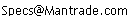Daily Speculations The Web Site of Victor Niederhoffer & Laurel Kenner Dedicated to the scientific method, free markets, deflating ballyhoo, creating value, and laughter;  a forum for us to use our meager abilities to make the world of specinvestments a better place.

Write to us at:(address is not clickable)

8/21/04:
Victor Niederhoffer: What the Average Person Sees in a Chart

Let’s take a random series where today's price is equal to yesterday's price plus or minus a random independent (with no memory and no drift) step size. Such a series is called a random walk.

The average variance of the position of the (drunk, particle or price) in a random walk after n steps is the number of steps times the square of the step size.

Thus, if the average step plus or minus in a day is 10 points per day (about 1% in the S&P) then after 16 days we can expect that the variance will be 16 x 100. The standard variation (standard deviation) will then be 40 or 4%.

Half of the time the move away from 0 will be 2.5% or more in such a case and 1/4 of the time the move away from 0 will be 4.5% or more.

(One-quarter of the area of a normal curve is 3/5 or more standard deviation away from the mean (of 0) and 1/8 of the area of a normal curve is 1.1 or more standard deviations away from the mean (of zero in this case).

This simple statistical concomitant of the way things vary over time, and the failure to appreciate it by all but the highest of trained simulators or statisticians, is the reason that so many think that there are trends in charts.

Indeed a move of 2.5 %, i.e., a trend of 2.5% in one direction, occurs about half the time by chance in any randomly chosen three-week interval (about 16 days) of a daily chart.

But let's suppose, as is one's wont, that in looking at a chart, one looks at the move of 10 separate three-week intervals and selects the three-week change that looks to be the maximum of the 10. You'd expect it to be more than 2.5% because you've selecting a maximum of something that normally is 2.5%. Indeed, if the moves are distributed according to the normal distribution (a good working model), then the maximum of 10 such moves (which can be found in any book or table on order statistics), is expected to be 1.5 times the average move of 2.5%.

Thus, we expect that in a garden variety chart where the average daily change is about 1%, when we look casually at 10 separate three-week changes, (let’s say, a six-month chart) and select the highest of these three-week changes, that on average such change will show a move of 4.%. It's this 4 % move that the chart person or trendist intuitively takes away from the chart that falsely gives one the impression that something unusual has transpired.